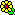## the results of sample size based on PowerTOST [Software]

Hi,

I have a naive question about the results of sample size based on SampleN.TOST, sampleN.NTIDFDA, sampleN.HVNTID etc.

For example,
sampleN.RSABE(CV=0.3) ++++++++ Reference scaled ABE crit. +++++++++            Sample size estimation --------------------------------------------- Study design:  2x3x3 (partial replicate) log-transformed data (multiplicative model) 1e+05 studies for each step simulated. alpha  = 0.05, target power = 0.8 CVw(T) = 0.3; CVw(R) = 0.3 True ratio = 0.9 ABE limits / PE constraints = 0.8 ... 1.25 FDA regulatory settings - CVswitch            = 0.3 - regulatory constant = 0.8925742 - pe constraint applied Sample size search  n     power 39   0.75781 42   0.78122 45   0.80344

I want to know if the target power is 0.8, the sample size is 45 for each group or for whole study.

The other question,
when we apply power.TOST, the sample size we need to fill in should be number in each group or whole study.

Third question,I am confused about the relationship between power and period. Are the power calculation method applied in 2*2, 3*3 and 2*4 designs the same?For example, if I have the result like this of the study design as T-S-R, could I use the same method to calculate power separately for T-R and S-R? Should I get two power as the result? And, what about parallel design?Thanks in advance!Ing. Helmut Schütz